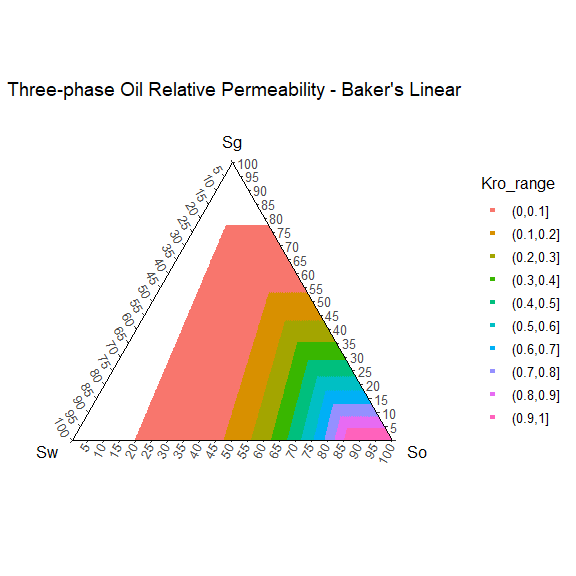# Rrelperm

Rrelperm generates relative permeability values for two- and/or three-phase mixtures of water and hydrocarbons. It estimates the two-phase relative permeabilities for the water-oil and gas-liquid systems in a tabular format using the modified Brooks-Corey correlation (Brooks & Corey, 1964). It calculates the three-phase relative permeabilities using the modified Stone I, Stone II, and Baker’s linear models (Baker, 1988; Fayers & Matthews, 1984; Stone, 1970).

## Relative Permeability Parameters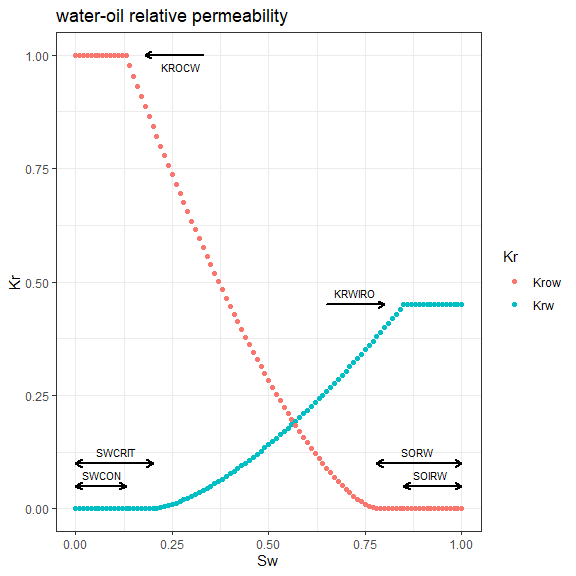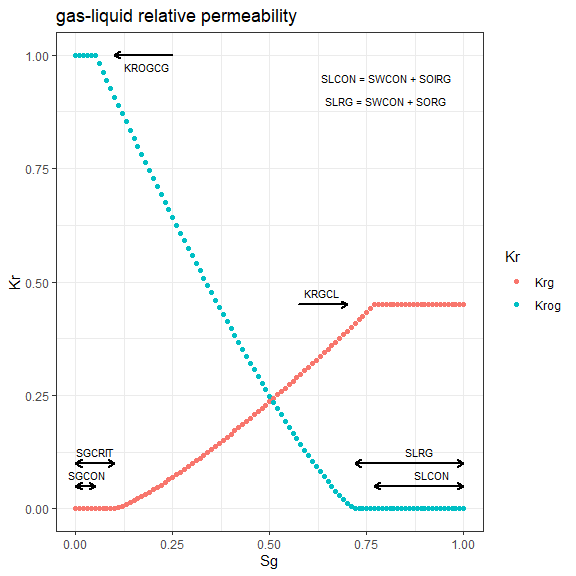## kr2p_ow() arguments

• SWCON: connate water saturation, fraction.
• SWCRIT: critical water saturation, fraction.
• SOIRW: irreducible oil saturation, fraction.
• SORW: residual oil saturation, fraction.
• KRWIRO: water relative permeability at irreducible oil.
• KROCW: oil relative permeability at connate water.
• NW: exponent term for calculating krw.
• NOW: exponent term for calculating krow.
• NP: number of saturation points in the table, the maximum acceptable value is 501.

## kr2p_gl() arguments

• SWCON: connate water saturation, fraction.
• SOIRG: irreducible oil saturation, fraction.
• SORG: residual oil saturation, fraction.
• SGCON: connate gas saturation, fraction.
• SGCRIT: critical gas saturation, fraction.
• KRGCL: gas relative permeability at connate liquid.
• KROGCG: oil relative permeability at connate gas.
• NG: exponent term for calculating krg.
• NOG: exponent term for calculating krog.
• NP: number of saturation points in the table, the maximum acceptable value is 501.

## kr3p_StoneI_So() arguments

• SWCON: connate water saturation, fraction.
• SWCRIT: critical water saturation, fraction.
• SOIRW: irreducible oil saturation, fraction.
• SORW: residual oil saturation, fraction.
• SOIRG: irreducible oil saturation, fraction.
• SORG: residual oil saturation, fraction.
• SGCON: connate gas saturation, fraction.
• SGCRIT: critical gas saturation, fraction.
• KRWIRO: water relative permeability at irreducible oil.
• KROCW: oil relative permeability at connate water.
• KRGCL: gas relative permeability at connate liquid.
• KROGCG: oil relative permeability at connate gas.
• NW: exponent term for calculating krw.
• NOW: exponent term for calculating krow.
• NG: exponent term for calculating krg.
• NOG: exponent term for calculating krog.
• NP: number of saturation points in the two-phase relative permeability tables, the maximum acceptable value is 501. The number of data points in the three-phase relative permeability table is (0.5 * NP * (NP + 1)).

## kr3p_StoneI_SwSg() arguments

• SWCON: connate water saturation, fraction.
• SWCRIT: critical water saturation, fraction.
• SOIRW: irreducible oil saturation, fraction.
• SORW: residual oil saturation, fraction.
• SOIRG: irreducible oil saturation, fraction.
• SORG: residual oil saturation, fraction.
• SGCON: connate gas saturation, fraction.
• SGCRIT: critical gas saturation, fraction.
• KRWIRO: water relative permeability at irreducible oil.
• KROCW: oil relative permeability at connate water.
• KRGCL: gas relative permeability at connate liquid.
• KROGCG: oil relative permeability at connate gas.
• NW: exponent term for calculating krw.
• NOW: exponent term for calculating krow.
• NG: exponent term for calculating krg.
• NOG: exponent term for calculating krog.
• NP: number of saturation points in the two-phase relative permeability tables, the maximum acceptable value is 501. The number of data points in the three-phase relative permeability table is (0.5 * NP * (NP + 1)).

## kr3p_StoneII_So() arguments

• SWCON: connate water saturation, fraction.
• SWCRIT: critical water saturation, fraction.
• SOIRW: irreducible oil saturation, fraction.
• SORW: residual oil saturation, fraction.
• SOIRG: irreducible oil saturation, fraction.
• SORG: residual oil saturation, fraction.
• SGCON: connate gas saturation, fraction.
• SGCRIT: critical gas saturation, fraction.
• KRWIRO: water relative permeability at irreducible oil.
• KROCW: oil relative permeability at connate water.
• KRGCL: gas relative permeability at connate liquid.
• KROGCG: oil relative permeability at connate gas.
• NW: exponent term for calculating krw.
• NOW: exponent term for calculating krow.
• NG: exponent term for calculating krg.
• NOG: exponent term for calculating krog.
• NP: number of saturation points in the two-phase relative permeability tables, the maximum acceptable value is 501. The number of data points in the three-phase relative permeability table is (0.5 * NP * (NP + 1)).

## kr3p_StoneII_SwSg() arguments

• SWCON: connate water saturation, fraction.
• SWCRIT: critical water saturation, fraction.
• SOIRW: irreducible oil saturation, fraction.
• SORW: residual oil saturation, fraction.
• SOIRG: irreducible oil saturation, fraction.
• SORG: residual oil saturation, fraction.
• SGCON: connate gas saturation, fraction.
• SGCRIT: critical gas saturation, fraction.
• KRWIRO: water relative permeability at irreducible oil.
• KROCW: oil relative permeability at connate water.
• KRGCL: gas relative permeability at connate liquid.
• KROGCG: oil relative permeability at connate gas.
• NW: exponent term for calculating krw.
• NOW: exponent term for calculating krow.
• NG: exponent term for calculating krg.
• NOG: exponent term for calculating krog.
• NP: number of saturation points in the two-phase relative permeability tables, the maximum acceptable value is 501. The number of data points in the three-phase relative permeability table is (0.5 * NP * (NP + 1)).

## kr3p_Baker() arguments

• SWCON: connate water saturation, fraction.
• SWCRIT: critical water saturation, fraction.
• SOIRW: irreducible oil saturation, fraction.
• SORW: residual oil saturation, fraction.
• SOIRG: irreducible oil saturation, fraction.
• SORG: residual oil saturation, fraction.
• SGCON: connate gas saturation, fraction.
• SGCRIT: critical gas saturation, fraction.
• KRWIRO: water relative permeability at irreducible oil.
• KROCW: oil relative permeability at connate water.
• KRGCL: gas relative permeability at connate liquid.
• KROGCG: oil relative permeability at connate gas.
• NW: exponent term for calculating krw.
• NOW: exponent term for calculating krow.
• NG: exponent term for calculating krg.
• NOG: exponent term for calculating krog.
• NP: number of saturation points in the two-phase relative permeability tables, the maximum acceptable value is 501. The number of data points in the three-phase relative permeability table is (0.5 * NP * (NP + 1)).

## Installation

The Rrelperm can be installed from CRAN with:

install.packages("Rrelperm")

## Two-phase Relative Permeability Examples

#### Example 1:

library(Rrelperm)
library(ggplot2)
library(magrittr)
library(tidyr)

rel_perm_ow <- kr2p_ow(SWCON = 0.13, SWCRIT = 0.13, SOIRW = 0.20, SORW = 0.2,
KRWIRO = 0.45, KROCW = 1, NW = 4.25, NOW = 2.5, NP = 101)

rel_perm_ow_df <- as.data.frame(rel_perm_ow)
colnames(rel_perm_ow_df) <- c("Sw", "So", "Krw", "Krow")

#>      Sw   So Krw Krow
#> 1  0.00 1.00   0    1
#> 2  0.01 0.99   0    1
#> 3  0.02 0.98   0    1
#> 4  0.03 0.97   0    1
#> 5  0.04 0.96   0    1
#> 6  0.05 0.95   0    1
#> 7  0.06 0.94   0    1
#> 8  0.07 0.93   0    1
#> 9  0.08 0.92   0    1
#> 10 0.09 0.91   0    1

p1 <- rel_perm_ow_df %>% tidyr::pivot_longer(cols = c(Krw, Krow), values_to = "value",
names_to = "Kr") %>%
ggplot(aes(x = Sw, y = value, col = Kr)) +
geom_point() +
labs(title = "water-oil relative permeability", x = "Sw", y = "Kr") +
theme_bw()

p1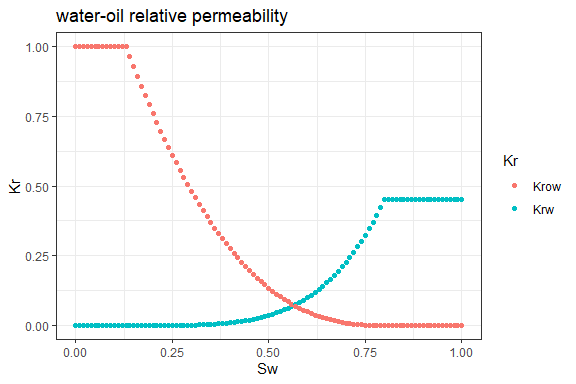#### Example 2:

library(Rrelperm)
library(ggplot2)
library(magrittr)

rel_perm_gl <- kr2p_gl(SWCON = 0.13, SOIRG = 0.12, SORG = 0.12, SGCON = 0.05,
SGCRIT = 0.05, KRGCL = 0.35, KROGCG = 1, NG = 4.25, NOG = 2.5,
NP = 101)

rel_perm_gl_df <- as.data.frame(rel_perm_gl)
colnames(rel_perm_gl_df) <- c("Sg", "Sl", "Krg", "Krog")

#>     Sg   Sl Krg Krog
#> 1 0.00 1.00   0    1
#> 2 0.01 0.99   0    1
#> 3 0.02 0.98   0    1
#> 4 0.03 0.97   0    1
#> 5 0.04 0.96   0    1
#> 6 0.05 0.95   0    1

p2 <- rel_perm_gl_df %>% tidyr::pivot_longer(cols = c(Krg, Krog), values_to = "value",
names_to = "Kr") %>%
ggplot(aes(x = Sg, y = value, col = Kr)) +
geom_point() +
labs(title = "gas-liquid relative permeability", x = "Sg", y = "Kr") +
theme_bw()

p2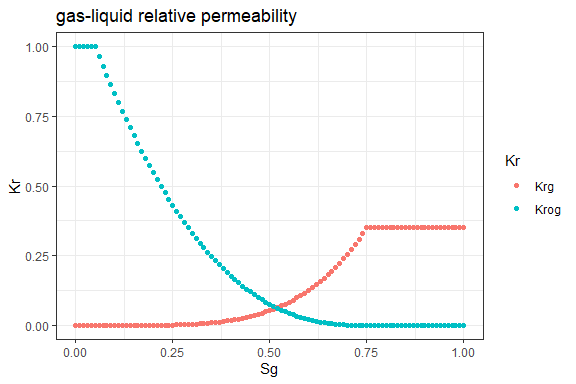## Three-phase Relative Permeability Examples

#### Example 1:

library(Rrelperm)
library(ggplot2)
library(ggtern)
#> Registered S3 methods overwritten by 'ggtern':
#>   method           from
#>   grid.draw.ggplot ggplot2
#>   plot.ggplot      ggplot2
#>   print.ggplot     ggplot2
#> --
#> Remember to cite, run citation(package = 'ggtern') for further info.
#> --
#>
#> Attaching package: 'ggtern'
#> The following objects are masked from 'package:ggplot2':
#>
#>     aes, annotate, ggplot, ggplotGrob, ggplot_build, ggplot_gtable,
#>     ggsave, layer_data, theme_bw, theme_classic, theme_dark,
#>     theme_gray, theme_light, theme_linedraw, theme_minimal, theme_void
library(magrittr)

rel_perm_owg_st_i_swsg <- kr3p_StoneI_SwSg(SWCON = 0.13, SWCRIT = 0.13, SOIRW = 0.20,
SORW = 0.2, SOIRG = 0.1, SORG = 0.1, SGCON = 0,
SGCRIT = 0, KRWIRO = 0.45, KROCW = 1, KRGCL = 0.35,
NW = 4.25, NOW = 2.5, NG = 3, NOG = 2,
NP = 501)

rel_perm_owg_st_i_swsg <- as.data.frame(rel_perm_owg_st_i_swsg)
colnames(rel_perm_owg_st_i_swsg) <- c("Sw", "Sg", "So", "Kro")

#>    Sw    Sg    So       Kro
#> 1   0 0.000 1.000 1.0000000
#> 2   0 0.002 0.998 0.9952956
#> 3   0 0.004 0.996 0.9906019
#> 4   0 0.006 0.994 0.9859188
#> 5   0 0.008 0.992 0.9812464
#> 6   0 0.010 0.990 0.9765846
#> 7   0 0.012 0.988 0.9719336
#> 8   0 0.014 0.986 0.9672932
#> 9   0 0.016 0.984 0.9626635
#> 10  0 0.018 0.982 0.9580444

rel_perm_owg_st_i_swsg$Kro_range <- cut(rel_perm_owg_st_i_swsg$Kro, breaks = seq(0,1, by = 0.1),
right = TRUE, include.lowest = FALSE)

rel_perm_owg_st_i_swsg_oil <- rel_perm_owg_st_i_swsg[rel_perm_owg_st_i_swsg$Kro > 0, ] rel_perm_owg_st_i_swsg_oil <- droplevels(rel_perm_owg_st_i_swsg_oil) p3 <- ggtern(data = rel_perm_owg_st_i_swsg_oil, aes(x = Sw, y = Sg, z = So, value = Kro)) + geom_point(aes(value = Kro, fill = Kro_range, color = Kro_range), size = 1, shape = 22) + scale_T_continuous(limits = c(0.0,1.0), breaks = seq(0,1,by = 0.05)) + scale_L_continuous(limits = c(0.0,1.0), breaks = seq(0,1,by = 0.05)) + scale_R_continuous(limits = c(0.0,1.0), breaks = seq(0,1,by = 0.05)) + ggtitle("Three-phase Oil Relative Permeability - STONE I, SWSG") + theme_classic() #> Warning: Ignoring unknown aesthetics: value p3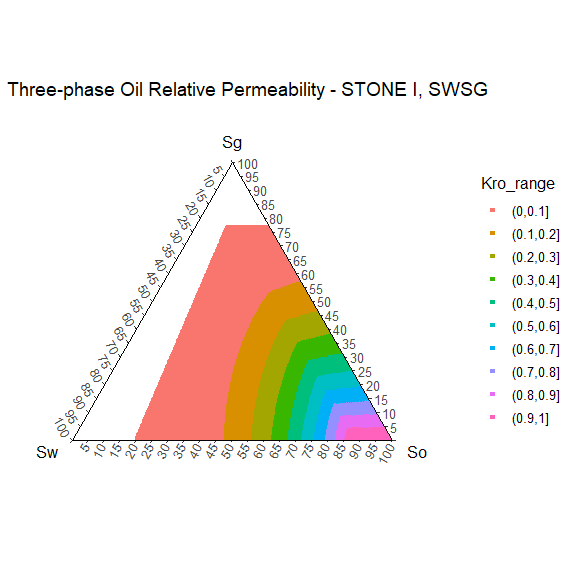#### Example 2: library(Rrelperm) library(ggplot2) library(ggtern) library(magrittr) rel_perm_owg_st_i_so <- kr3p_StoneI_So(SWCON = 0.13, SWCRIT = 0.13, SOIRW = 0.20, SORW = 0.2, SOIRG = 0.1, SORG = 0.1, SGCON = 0, SGCRIT = 0, KRWIRO = 0.45, KROCW = 1, KRGCL = 0.35, NW = 4.25, NOW = 2.5, NG = 3, NOG = 2, NP = 501) rel_perm_owg_st_i_so <- as.data.frame(rel_perm_owg_st_i_so) colnames(rel_perm_owg_st_i_so) <- c("Sw", "Sg", "So", "Kro") head(rel_perm_owg_st_i_so, 10) #> Sw Sg So Kro #> 1 0 0.000 1.000 1 #> 2 0 0.002 0.998 1 #> 3 0 0.004 0.996 1 #> 4 0 0.006 0.994 1 #> 5 0 0.008 0.992 1 #> 6 0 0.010 0.990 1 #> 7 0 0.012 0.988 1 #> 8 0 0.014 0.986 1 #> 9 0 0.016 0.984 1 #> 10 0 0.018 0.982 1 rel_perm_owg_st_i_so$Kro_range <- cut(rel_perm_owg_st_i_so$Kro, breaks = seq(0,1, by = 0.1), right = TRUE, include.lowest = FALSE) rel_perm_owg_st_i_so_oil <- rel_perm_owg_st_i_so[rel_perm_owg_st_i_so$Kro > 0, ]

rel_perm_owg_st_i_so_oil <- droplevels(rel_perm_owg_st_i_so_oil)

p4 <- ggtern(data = rel_perm_owg_st_i_so_oil, aes(x = Sw, y = Sg, z = So, value = Kro)) +
geom_point(aes(value = Kro, fill = Kro_range, color = Kro_range), size = 1, shape = 22) +
scale_T_continuous(limits = c(0.0,1.0), breaks = seq(0,1,by = 0.05)) +
scale_L_continuous(limits = c(0.0,1.0), breaks = seq(0,1,by = 0.05)) +
scale_R_continuous(limits = c(0.0,1.0), breaks = seq(0,1,by = 0.05)) +
ggtitle("Three-phase Oil Relative Permeability - STONE I, SO") +
theme_classic()
#> Warning: Ignoring unknown aesthetics: value

p4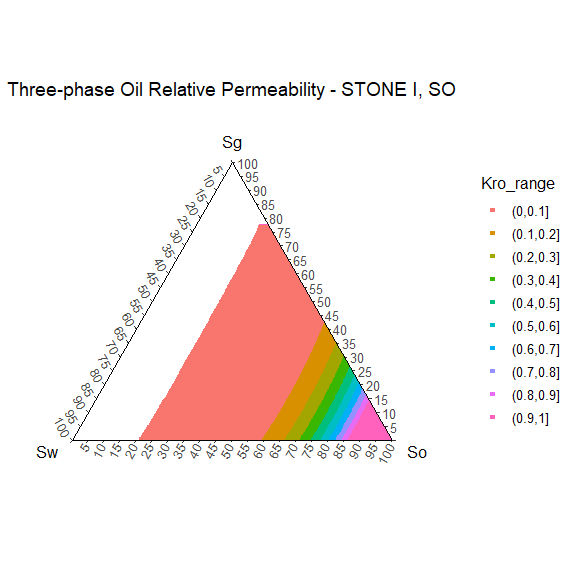#### Example 3:

library(Rrelperm)
library(ggplot2)
library(ggtern)
library(magrittr)

rel_perm_owg_st_ii_swsg <- kr3p_StoneII_SwSg(SWCON = 0.13, SWCRIT = 0.13, SOIRW = 0.20,
SORW = 0.2, SOIRG = 0.1, SORG = 0.1, SGCON = 0,
SGCRIT = 0, KRWIRO = 0.45, KROCW = 1, KRGCL = 0.35,
NW = 4.25, NOW = 2.5, NG = 3, NOG = 2,
NP = 501)

rel_perm_owg_st_ii_swsg <- as.data.frame(rel_perm_owg_st_ii_swsg)
colnames(rel_perm_owg_st_ii_swsg) <- c("Sw", "Sg", "So", "Kro")

#>    Sw    Sg    So       Kro
#> 1   0 0.000 1.000 1.0000000
#> 2   0 0.002 0.998 0.9948119
#> 3   0 0.004 0.996 0.9896374
#> 4   0 0.006 0.994 0.9844763
#> 5   0 0.008 0.992 0.9793287
#> 6   0 0.010 0.990 0.9741946
#> 7   0 0.012 0.988 0.9690740
#> 8   0 0.014 0.986 0.9639669
#> 9   0 0.016 0.984 0.9588733
#> 10  0 0.018 0.982 0.9537932

rel_perm_owg_st_ii_swsg$Kro_range <- cut(rel_perm_owg_st_ii_swsg$Kro, breaks = seq(0,1, by = 0.1),
right = TRUE, include.lowest = FALSE)

rel_perm_owg_st_ii_swsg_oil <- rel_perm_owg_st_ii_swsg[rel_perm_owg_st_ii_swsg$Kro > 0, ] rel_perm_owg_st_ii_swsg_oil <- droplevels(rel_perm_owg_st_ii_swsg_oil) p5 <- ggtern(data = rel_perm_owg_st_ii_swsg_oil, aes(x = Sw, y = Sg, z = So, value = Kro)) + geom_point(aes(value = Kro, fill = Kro_range, color = Kro_range), size = 1, shape = 22) + scale_T_continuous(limits = c(0.0,1.0), breaks = seq(0,1,by = 0.05)) + scale_L_continuous(limits = c(0.0,1.0), breaks = seq(0,1,by = 0.05)) + scale_R_continuous(limits = c(0.0,1.0), breaks = seq(0,1,by = 0.05)) + ggtitle("Three-phase Oil Relative Permeability - STONE II, SWSG") + theme_classic() #> Warning: Ignoring unknown aesthetics: value p5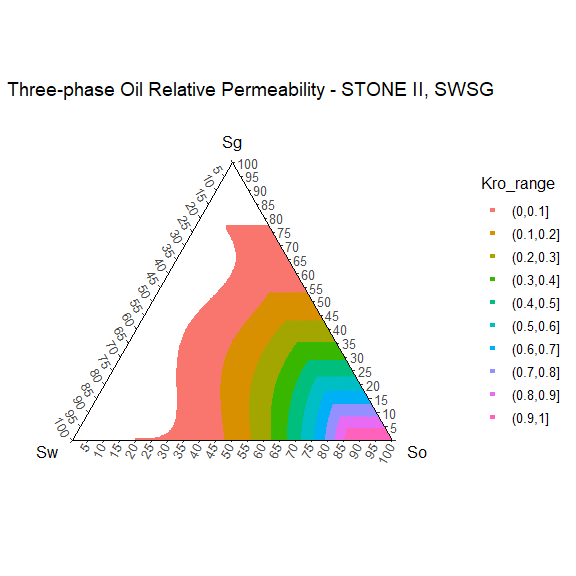#### Example 4: library(Rrelperm) library(ggplot2) library(ggtern) library(magrittr) rel_perm_owg_st_ii_so <- kr3p_StoneII_So(SWCON = 0.13, SWCRIT = 0.13, SOIRW = 0.20, SORW = 0.2, SOIRG = 0.1, SORG = 0.1, SGCON = 0, SGCRIT = 0, KRWIRO = 0.45, KROCW = 1, KRGCL = 0.35, NW = 4.25, NOW = 2.5, NG = 3, NOG = 2, NP = 501) rel_perm_owg_st_ii_so <- as.data.frame(rel_perm_owg_st_ii_so) colnames(rel_perm_owg_st_ii_so) <- c("Sw", "Sg", "So", "Kro") head(rel_perm_owg_st_ii_so, 10) #> Sw Sg So Kro #> 1 0 0.000 1.000 1 #> 2 0 0.002 0.998 1 #> 3 0 0.004 0.996 1 #> 4 0 0.006 0.994 1 #> 5 0 0.008 0.992 1 #> 6 0 0.010 0.990 1 #> 7 0 0.012 0.988 1 #> 8 0 0.014 0.986 1 #> 9 0 0.016 0.984 1 #> 10 0 0.018 0.982 1 rel_perm_owg_st_ii_so$Kro_range <- cut(rel_perm_owg_st_ii_so$Kro, breaks = seq(0,1, by = 0.1), right = TRUE, include.lowest = FALSE) rel_perm_owg_st_ii_so_oil <- rel_perm_owg_st_ii_so[rel_perm_owg_st_ii_so$Kro > 0, ]

rel_perm_owg_st_ii_so_oil <- droplevels(rel_perm_owg_st_ii_so_oil)

p6 <- ggtern(data = rel_perm_owg_st_ii_so_oil, aes(x = Sw, y = Sg, z = So, value = Kro)) +
geom_point(aes(value = Kro, fill = Kro_range, color = Kro_range), size = 1, shape = 22) +
scale_T_continuous(limits = c(0.0,1.0), breaks = seq(0,1,by = 0.05)) +
scale_L_continuous(limits = c(0.0,1.0), breaks = seq(0,1,by = 0.05)) +
scale_R_continuous(limits = c(0.0,1.0), breaks = seq(0,1,by = 0.05)) +
ggtitle("Three-phase Oil Relative Permeability - STONE II, SO") +
theme_classic()
#> Warning: Ignoring unknown aesthetics: value

p6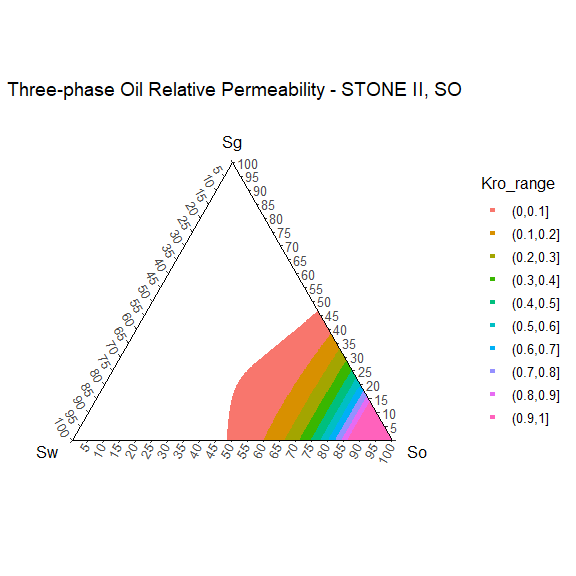#### Example 5:

library(Rrelperm)
library(ggplot2)
library(ggtern)
library(magrittr)

rel_perm_owg_bk <- kr3p_Baker(SWCON = 0.13, SWCRIT = 0.13, SOIRW = 0.20,
SORW = 0.2, SOIRG = 0.1, SORG = 0.1, SGCON = 0,
SGCRIT = 0, KRWIRO = 0.45, KROCW = 1, KRGCL = 0.35,
NW = 4.25, NOW = 2.5, NG = 3, NOG = 2,
NP = 501)

rel_perm_owg_bk <- as.data.frame(rel_perm_owg_bk)
colnames(rel_perm_owg_bk) <- c("Sw", "Sg", "So", "Kro")

#>    Sw    Sg    So       Kro
#> 1   0 0.000 1.000 1.0000000
#> 2   0 0.002 0.998 0.9948119
#> 3   0 0.004 0.996 0.9896374
#> 4   0 0.006 0.994 0.9844763
#> 5   0 0.008 0.992 0.9793287
#> 6   0 0.010 0.990 0.9741946
#> 7   0 0.012 0.988 0.9690740
#> 8   0 0.014 0.986 0.9639669
#> 9   0 0.016 0.984 0.9588733
#> 10  0 0.018 0.982 0.9537932

rel_perm_owg_bk$Kro_range <- cut(rel_perm_owg_bk$Kro, breaks = seq(0,1, by = 0.1),
right = TRUE, include.lowest = FALSE)

rel_perm_owg_bk_oil <- rel_perm_owg_bk[rel_perm_owg_bk\$Kro > 0, ]

rel_perm_owg_bk_oil <- droplevels(rel_perm_owg_bk_oil)

p7 <- ggtern(data = rel_perm_owg_bk_oil, aes(x = Sw, y = Sg, z = So)) +
geom_point(aes(value = Kro, fill = Kro_range, color = Kro_range), size = 1, shape = 22) +
scale_T_continuous(limits = c(0.0,1.0), breaks = seq(0,1,by = 0.05)) +
scale_L_continuous(limits = c(0.0,1.0), breaks = seq(0,1,by = 0.05)) +
scale_R_continuous(limits = c(0.0,1.0), breaks = seq(0,1,by = 0.05)) +
ggtitle("Three-phase Oil Relative Permeability - Baker's Linear") +
theme_classic()
#> Warning: Ignoring unknown aesthetics: value

p7